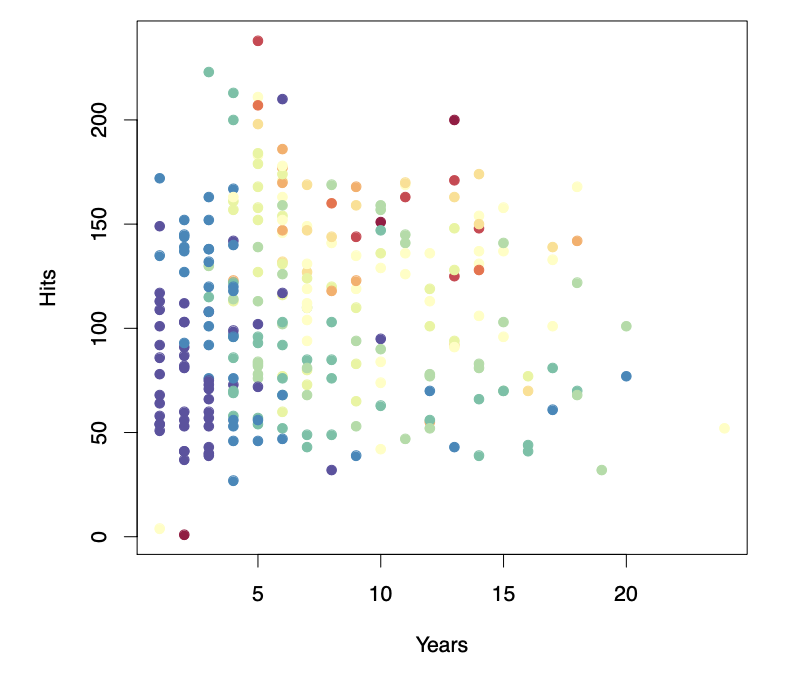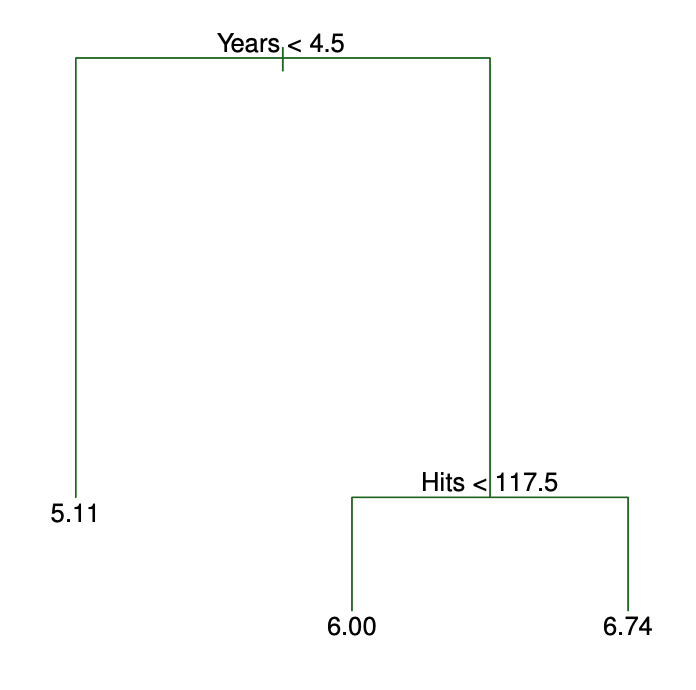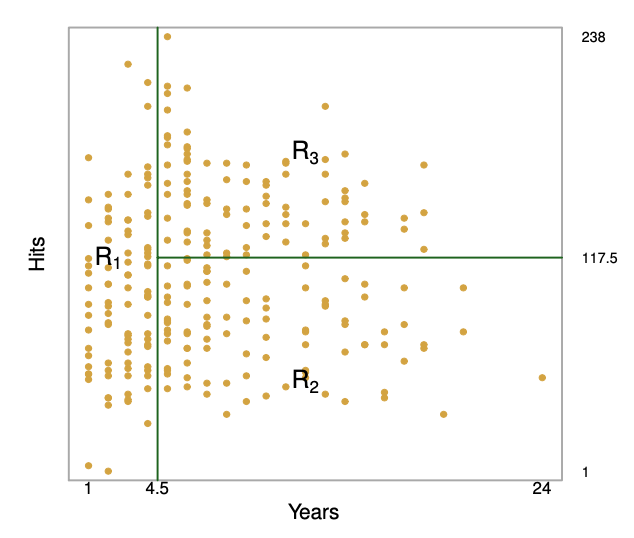## Tree-based Methods

• Here we describe tree-based methods for regression and classification

• These involve stratifying or segmenting the predictor space into a number of simple regions

• Since the set of splitting rules used to segment the predictor space can be summarized in a tree, these types of approaches are known as decision-tree methods

• Tree-based methods are simple and useful for interpretation

• However they typically are not competitive with the best supervised learning approaches in terms of prediction accuracy

• Hence we also discuss bagging, random forests, and boosting. These methods grow multiple trees which are then combined to yield a single consensus prediction

• Combining a large number of trees can often result in dramatic improvements in prediction accuracy, at the expense of some loss interpretation

## The Basics of Decision Trees

• Decision trees can be applied to both regression and classification problems

• We first consider regression problems, and then move on to classification

• Example

• Baseball Salary Data: How would you stratify it?

• Salary is color-coded from low (blue, green) to high (yellow, red)• Decision tree for these data:• For the Hitters data, a regression tree for predicting the log salary of a baseball player, based on the number of years that he has played in the major leagues and the number of hits that he made in the previous year

• At a given internal node, the label (of the form $$X_j < t_k$$) indicates the left-hand branch emanating from that split, and the right-hand branch corresponds to $$X_j > t_k$$. For instance, the split at the top of the tree results in two large branches. The left-hand branch corresponds to Years$<$$4.5, and the right-hand branch corresponds to Years$$$4.5

• The tree has two internal nodes and three terminal nodes, or leaves. The number in each leaf is the mean of the response for the observations that fall there

• Results

• Overall, the tree stratifies or segments the players into three regions of predictor space:

• $$R_1 = {X | Years < 4.5}, R_2 = {X | Years \geq 4.5, Hits < 117.5}, \ and \ R_3 = {X | Years \geq 4.5, Hits \geq 117.5}$$## Terminology for Trees

• In keeping with the tree analogy, the regions $$R_1, R_2, R_3$$ are known as terminal nodes

• Decision trees are typically drawn upside down, in the sense that the leaves are at the bottom of the tree

• The points along the tree where the predictor space is split are referred to as internal nodes

• In the hitters tree, the two internal nodes are indicated by the text $$Years < 4.5$$ and $$Hits < 117.5$$

## Interpretation of Results

• Years is the most important factor in determining Salary, and players with less experience earn lower salaries than more experienced players

• Given that a player is less experienced, the number of Hits that he made in the previous year seems to play little role in his Salary

• But among players who have been in the major leagues for five or more years, the number of Hits made in the previous year does affect Salary, and players who made more Hits last year tend to have higher salaries

• Surely an over-simplification, but compared to a regression model, it is easy to display, interpret and explain

## Details of the Tree-Building Process

• We divide the predictor space – that is the set of possible values for $$X_1, X_2, ... , X_p$$ – into $$J$$ distinct and non-overlapping regions, $$R_1, R_2, ... , R_j$$

• For every observation that falls into the region $$R_1$$, we make the same prediction, which is simply the mean of the response values for the training observations in $$R_1$$

• In theory, the regions could have any shape. However, we choose to divide the predictor space into high-dimensional rectangles, or boxes, for simplicity and for ease of interpretation of the resulting predictive model

• The goal is to find boxes $$R_1, R_2, ... , R_j$$ that minimize the RSS given by:

• $$\sum_{j=1}^{j}\sum_{i \in R_j}(y_i - \hat{y}_{R_j})^2$$

• Where $$\hat{y}_{R_j}$$ is the mean response for the training observation within the $$j_th$$ box
• Unfortunately, it is computationally infeasible to consider every possible partition of the feature space into $$J$$ boxes

• For this reason, we take a top-down, greedy approach that is known as recursive binary splitting

• The approach is top-down because it begins at the top of the tree and then successively splits the predictor space; each split is indicated via two new branches further down on the tree

• It is greedy because at each step of the tree-building process, the best split is made at that particular step, rather than looking ahead and picking a split that will lead to a better tree in some future step

• We first select the predictor $$X_j$$ and the cutpoint $$s$$ such that splitting the predictor space into the regions $${X|X_j < s}$$ and $${X|X_j \geq s}$$

• Next, we repeat the process, looking for the best predictor and best cutpoint in order to split the data further so as to minimize the RSS within each of the resulting regions

• However, this time, instead of splitting the entire predictor space, we split one of the two previously identified regions. We now have three regions

• Again, we look to split one of these three regions further, so as to minimize the RSS. The process continues until a stopping criterion is reached; for instance, we may continue until no region contains more than five observations

## Predictions

• We predict the response for a given test observation using the mean of the training observations in the region to which that test observation belongs

• A five-region example of this approach is shown in the next slide